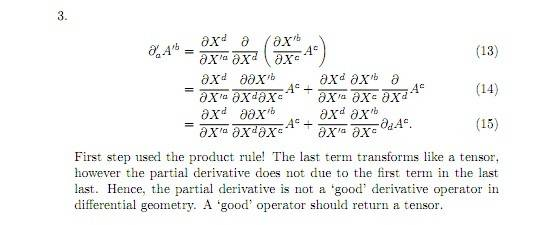# Coordinate transform of partial derivative

• ck99
However, in higher dimensions, the product rule would still apply and the double derivative would be written as \frac{\partial^2}{\partial x^2}

## Homework Statement

How does ∂aAb behave under coordinate transformations in special relativity? Work out ∂'aA'b

## The Attempt at a Solution

I have been given back the solution sheet to this problem, but I don't understand it. This is what I haveI get the first line, (13). We have treated the partial as a covariant vector, and written out the usual transform for it. The part within the brackets is the normal transform of a contravariant vector A.

Looking at (14), the text says we have used the product rule here. I am familiar with this in normal calculus, where if k(x) = f(x)g(x) then k'(x) = f'(x)g(x) + f(x)g'(x). If I apply that here, I would expect to see ∂'aAb + ∂aA'b

Written out long hand, this would be similar to (14). However, the solution has an extra ∂Xd/∂X'a in the second term, and that weird ∂∂X'b thing in the first term.

For the second term in (14) we have every item in (13) just multiplied together in a different order; and the first term in (14) is the same, but with two of those items conflated to form the ∂∂X'b strangeness. Are they multiplied together in some sense, or is this now a second order partial derivative term? What on Earth is going on? If someone could post up a clear rule for how to approach this type of problem it would be great, as it will keep cropping up in my course and I am totally clueless how else to tackle it.

My tutor advised me to take a look at the wikipedia link for the chain rule, but I can't see how that is relevant to this problem, although I see it is different in higher dimensions to what I am used to. I have also searched to see if the product rule is different in higher dimensions, but it seems to work just the same for vectors as for normal functions.

You're starting off with
$$\partial'_a A'^b = \frac{\partial X^d}{\partial X'^a} \frac{\partial}{\partial X^d} \left(\frac{\partial X'^b}{\partial X^c} A^c\right) = \frac{\partial X^d}{\partial X'^a} \left[\frac{\partial}{\partial X^d} \left(\frac{\partial X'^b}{\partial X^c} A^c\right)\right]$$You use the product rule to evaluate the term in the square brackets to get
$$\partial'_a A'^b = \frac{\partial X^d}{\partial X'^a} \left[ \left(\frac{\partial}{\partial X^d} \frac{\partial X'^b}{\partial X^c}\right) A^c + \frac{\partial X'^b}{\partial X^c} \left(\frac{\partial}{\partial X^d} A^c\right)\right]$$

Thanks for that Vela, I can follow your explanation although I am not sure why we do it that way instead of putting the terms in a different order or changing the position of the square brackets - probably some property of the partial operator which I am not familiar with. As long as I know how to do it, that is a victory!

Could you (or someone else) explain what the "double derivative" ∂∂X'b/∂Xd∂Xc means? It looks like it's the rate of change of a derivative, which should be a second-order derivative I think, but it's not written that way.

I'm not sure where your confusion lies because there's really no other way to do that calculation. It's just using the standard rules you learn in calculus about differentiating.

That term is a second-order derivative. In the "normal" notation, it would correspond go a term like $\frac{\partial^2}{\partial x\partial y}$ if c≠d, and to a term like $\frac{\partial^2}{\partial x^2}$ if c=d.